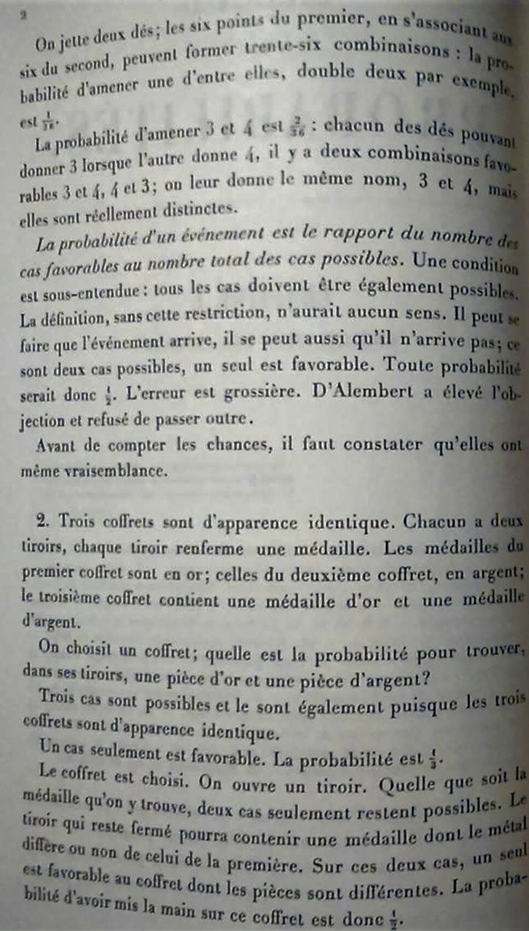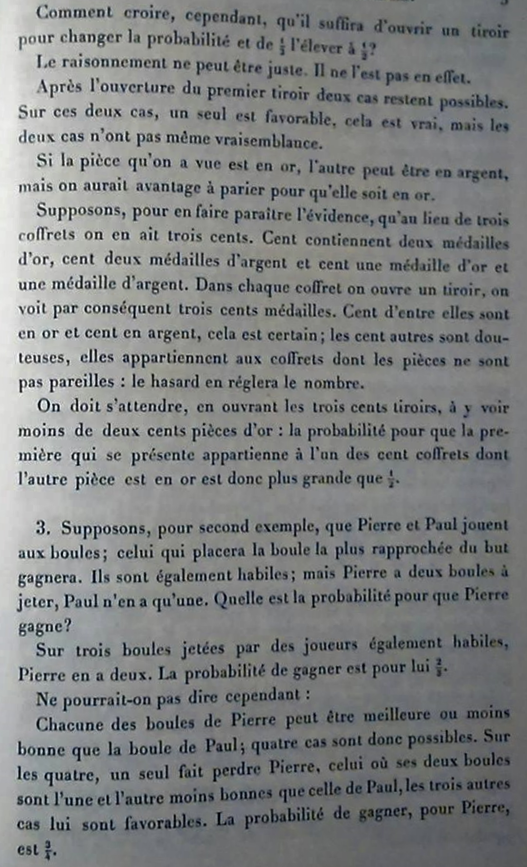# Literal and Liberal Translations of Bertrand’s Box Paradox

In his 1889 book “Calcul des Probabilités”, the French mathematician Joseph Bertrand (1822–1900) introduced a probability paradox that anticipates both the Monty Hall problem and the Three Prisoners problem. Below we first present a literal translation of Bertrand’s text, which unfortunately suffers from being somewhat unclear. We therefore follow it up with a more liberal translation, and end with a more concise description by Emile Borel (1965). The French original is presented at the end of this post.

# The Literal Translation

“Three boxes have an identical appearance. Each has two drawers and each drawer contains one coin. The coins of the first box are of gold; those of the second box are of silver; the third box contains one gold coin and one silver coin.

One chooses a box; what is the probability of finding, in its drawers, one gold coin and one silver coin?

There are three cases and these are equally possible because the three boxes have an identical appearance.

Only one case is favorable. The probability is 1/3.

A box is chosen. A drawer is opened. Whatever coin one finds, only two cases remain possible. The drawer that remains closed could contain a coin of which the metal either differs or not from that of the first. Of these two cases, one is favorable for the box of which the coins are different. The probability of laying one’s hand on that box is therefore 1/2.

However, how can it be that opening a drawer suffices to change the probability and increase it from 1/3 to 1/2?

The reasoning cannot be correct. And in fact it is not.

After opening the first drawer two cases remain possible. Out of these two cases, only one is favorable, that is true, but the two cases are not equally probable.

If the coin that one has seen is of gold, the other one can be of silver, but one stands to gain by betting that it is of gold.

Suppose, to make it obvious, that instead of three boxes one has three hundred. One hundred boxes contain two gold coins, one hundred contain two silver coins, and one hundred contain one gold coin and one silver coin. Of each box one opens a drawer and consequently one sees three hundred coins. A hundred of these are of gold and a hundred of silver, this is certain; the other hundred are uncertain, these belong to the boxes of which the coins are not the same: probability will determine the number.

One has to prepare, upon opening the three hundred drawers, to find fewer than two hundred gold coins: the probability that the first coin that presents itself belongs to one of the hundred boxes of which the other coin is of gold is therefore greater than 1/2.” (Bertrand, 1889, pp. 2–3; translated with assistance of Bianca van Rossum)

# Liberal Translation

“There are three identical-looking boxes. Each box has two drawers and each drawer contains one coin. In the first box, each drawer contains a gold coin; in the second, each drawer contains a silver coin; and in the third, one drawer contains a gold coin and the other contains a silver coin.

One of the three boxes is chosen at random. What is the probability of finding one gold coin and one silver coin?

The answer seems obvious: There are three equally possible cases. Only one case gives the required outcome (one coin of each type). Hence, the probability is 1/3.

However, now consider what happens if, after choosing the box, we open one of its drawers at random. Let’s say we see a gold coin. We now know that we did not get the box with two silver coins. We have chosen either the box with two gold coins, or the box with one gold and one silver coin. The drawer that we have not opened may therefore contain a gold coin or a silver coin, with a probability for either event of 1/2. But now consider the alternative scenario: the first drawer reveals a silver coin. The same reasoning again leads to a probability of 1/2 for the unopened drawer to contain either a gold coin or a silver coin. So regardless of whether the first drawer shows a gold coin or a silver coin—and it is certain to show one of the two—the probability of finding a non-matching coin in the second drawer is 1/2. We therefore conclude that the mere act of opening a drawer changes the probability, increasing it from 1/3 to 1/2.

The reasoning cannot be correct. And in fact it is not.

It is true that, after opening the first drawer and seeing a gold coin, two cases (gold-gold and gold-silver) remain possible. It is also true that only one of these two gives us the gold-silver combination, whose probability we were asked to find. But the crucial point here is that these two cases were not equally likely to have happened in the first place.

To make this clearer, imagine that instead of three boxes we have three hundred: A hundred contain two gold coins, a hundred contain two silver coins, and a hundred contain one gold coin and one silver coin. We open one drawer of each box, revealing a total of 300 coins. For the hundred “double-gold” and the hundred “double-silver” boxes, we know that we will always see a gold or a silver coin, respectively. For the other hundred boxes, those with a gold and a silver coin, the proportions will be determined by chance, but we will probably see about 50 of each. However, we know that of the roughly 150 gold coins we see, 100 of them are in a gold-gold box and only 50 are in a gold-silver box. There (50 out of 150) is our correct probability of 1/3.

You can also see that, if we were asked to choose one of the open boxes in which we see a gold coin and to bet on what color the other coin in that box is, we would be wise to bet on gold, because in two-thirds of cases (100 out of 150) we would be right. Again, this corresponds to the fact that one-third of the boxes in which we can see a gold coin in the open drawer have a silver coin in the other (closed) drawer, whereas two-thirds have a gold coin in the other drawer.” (Bertrand, 1889, pp. 2-3)

# Borel’s Version

Another French mathematician, Émile Borel (1871-1956), provides a more succinct explanation of the paradox in his 1965 book “Elements of the Theory of Probability”:

“Each of three chests has two drawers. The first chest has a gold coin in each drawer, the second chest has a gold coin in one drawer and a silver coin in the other drawer, while the third chest has a silver coin in each drawer. If one opens one of the drawers and finds a gold coin, what is the probability that the second drawer of this chest will also contain a gold coin?

The question reduces to the following: What is the probability that the drawer which was opened belongs to the first chest? Since three of the drawers contain gold coins, each has a probability of 1/3; the required probability is thus 2/3, since two of the three drawers belong to the first chest.” (Borel, 1965, p. 122).

Although correct, this solution does not elaborate on the paradoxical character of Bertrand’s problem, which in its core is also reminiscent of the two envelopes problem and the necktie paradox.

Finally, here we present the original French description:#### References

Bertrand, J. (1889). Calcul des Probabilités. Paris: Gauthier-Villars et Fils.

Borel, E. (1965). Elements of the Theory of Probability. Englewood Cliffs, NJ: Prentice-Hall.### Nick Brown

Nick Brown is a psychologist and researcher into scientific error, affiliated with Linnaeus University, Sweden.### Eric-Jan Wagenmakers

Eric-Jan (EJ) Wagenmakers is professor at the Psychological Methods Group at the University of Amsterdam.